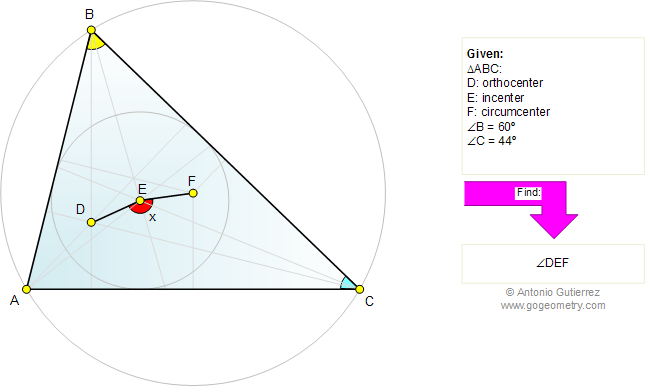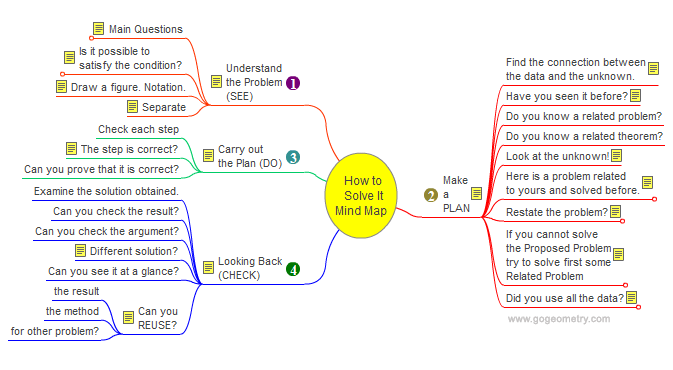Home Mind Map Problems All problems 661-670 Triangles Circles Incenter Circumcenter Orthocenter Solution/comment        By Antonio Gutierrez
 Problem 664. Triangle, 60 Degrees, Orthocenter, Incenter, Circumcenter, Angles. Level: High School, College. The figure shows a triangle ABC with angle B = 60 degrees, C = 44 degrees, D orthocenter, E incenter, and F circumcenter. Find the measure of angle DEF.Geometry problem solving Geometry problem solving is one of the most challenging skills for students to learn. When a problem requires auxiliary construction, the difficulty of the problem increases drastically, perhaps because deciding which construction to make is an ill-structured problem. By “construction,” we mean adding geometric figures (points, lines, planes) to a problem figure that wasn’t mentioned as "given."   How to Solve It, Interactive Mind Map George Pólya's 1945 book "How to Solve It, A new aspect of Mathematical Method", is a book describing methods of problem solving. It suggests the following steps when solving a mathematical problem: (1) First, you have to understand the problem. (2) After understanding, then make a plan. (3) Carry out the plan. (4) Look back on your work. How could it be better?    Mind Map Help. To see a note: Hover over a yellow note button. To Fold/Unfold: click a branch. To Pan: click and drag the map canvas.Recent Additions